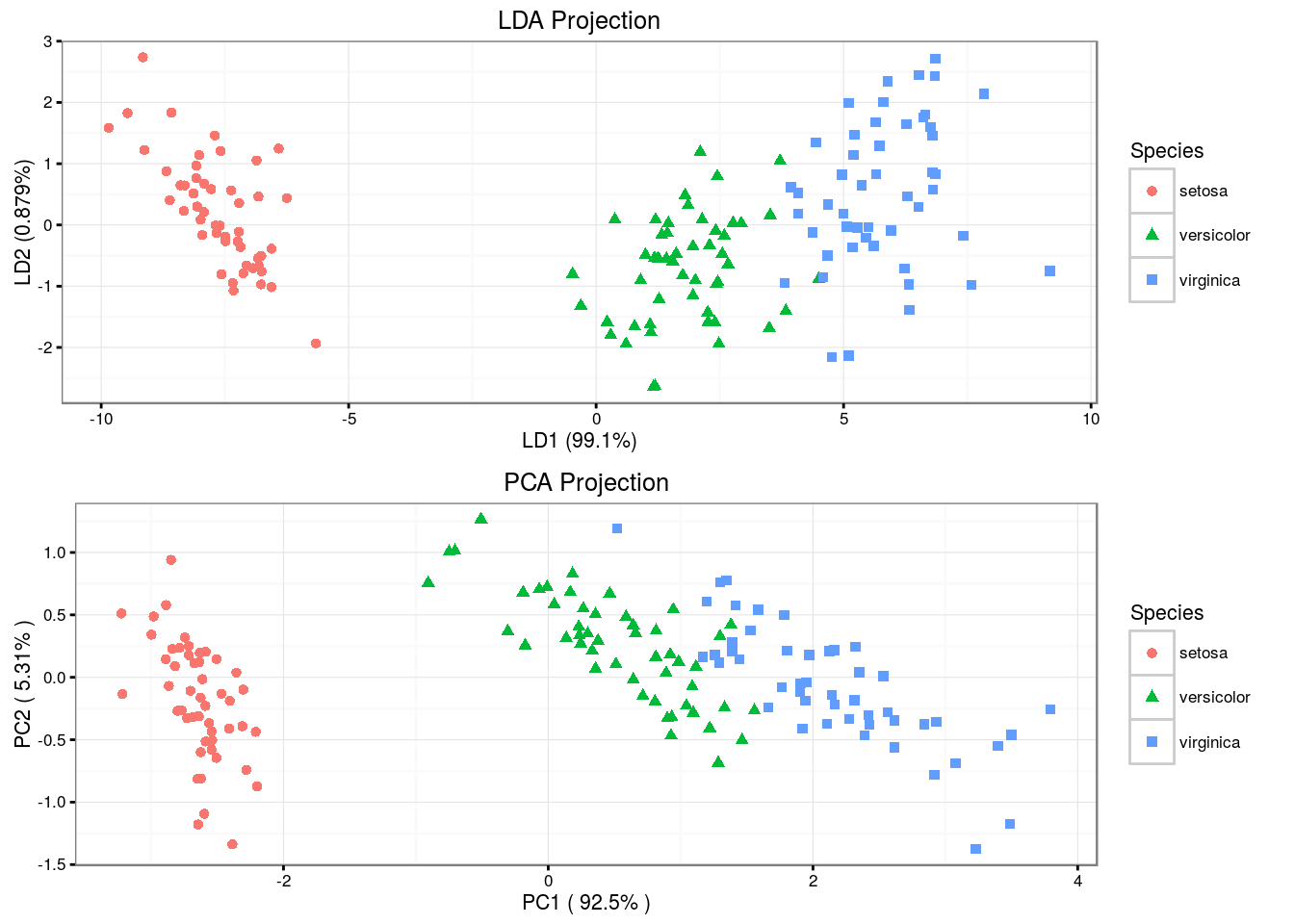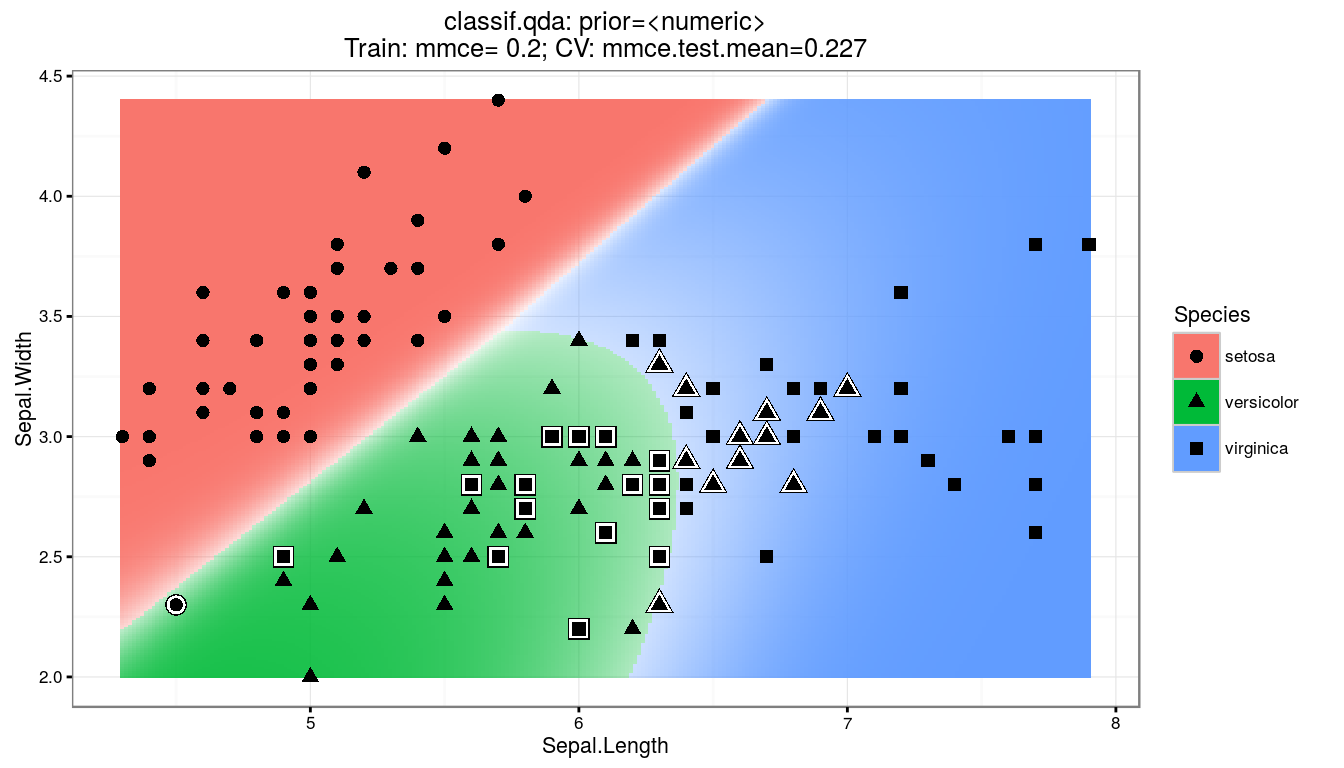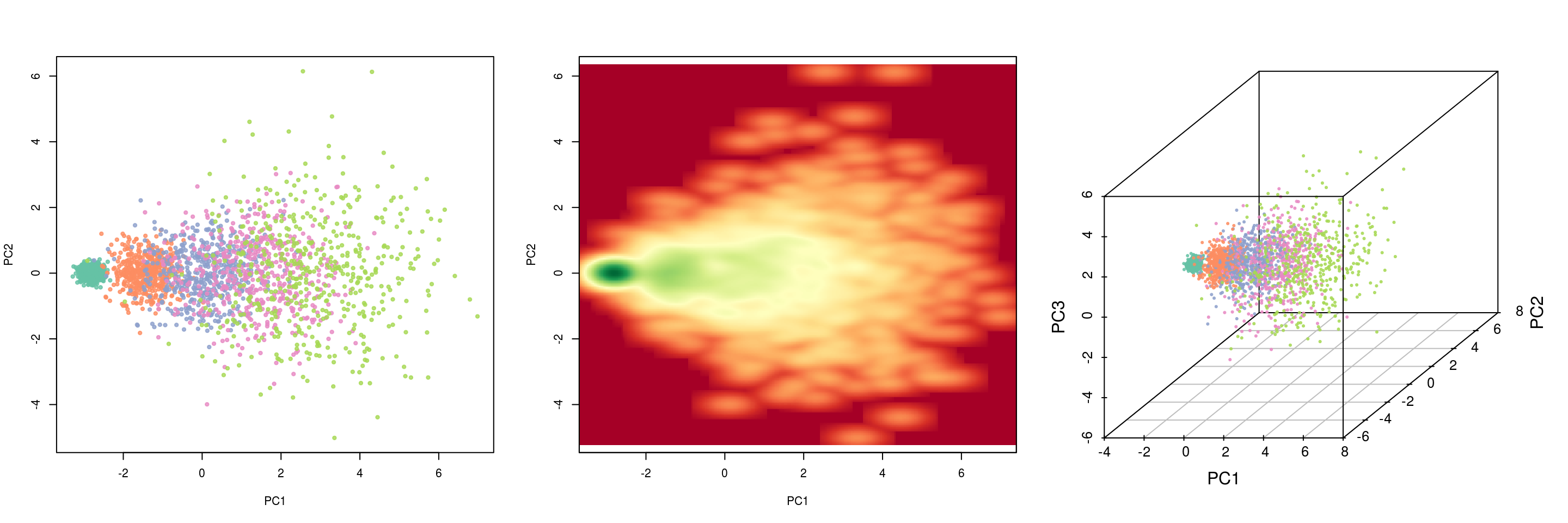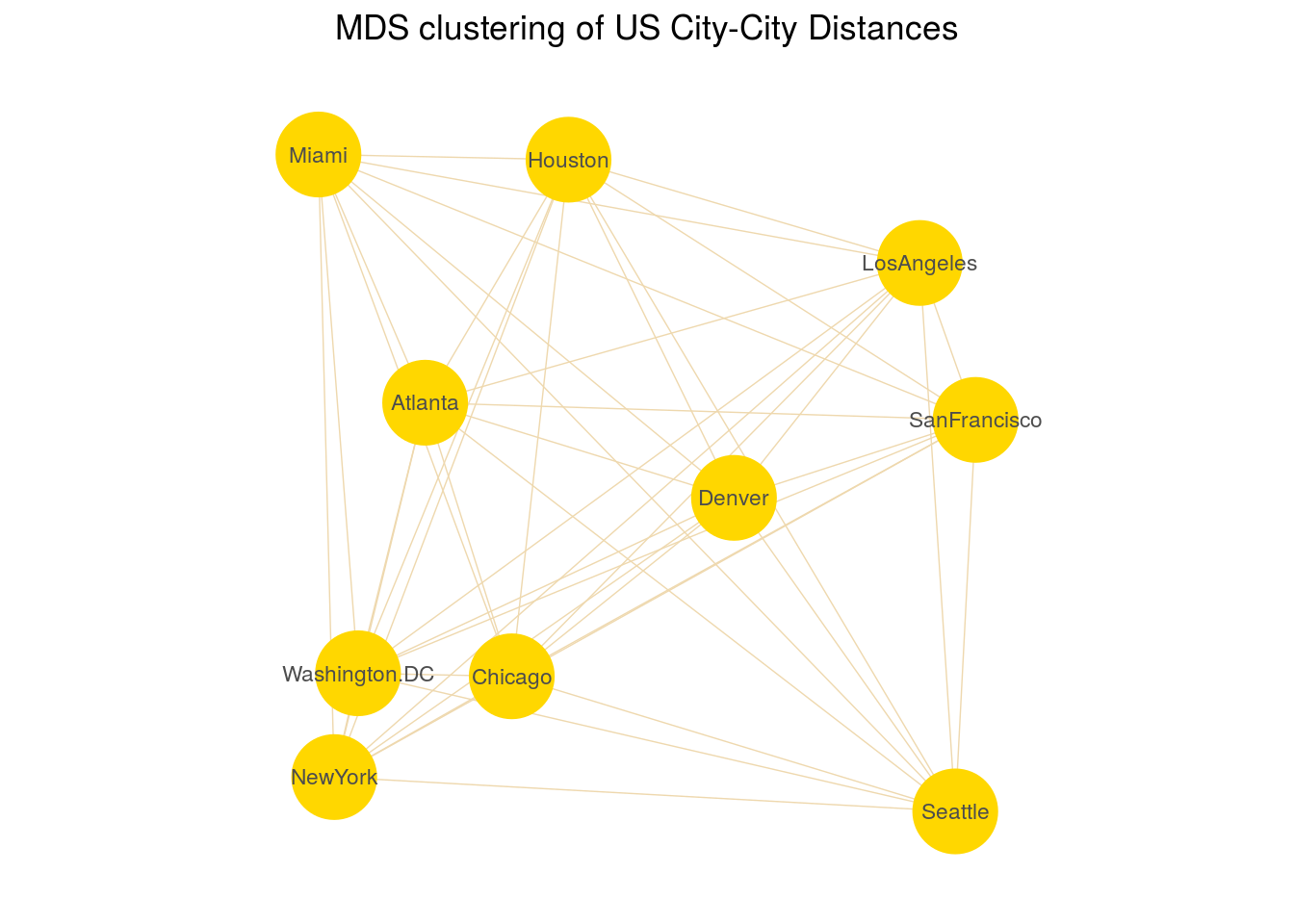## LDA

The LDA method approximates the Bayes classifier assuming that the $$p$$-dimensional random variable $$X$$ is drawn from a multivariate Gaussian distribution $${\mathcal N}(\mu_k ,\, \mathbf\Sigma)$$. The classifier assigns an observation $$X = x$$ to the class for which $\hat\delta_k(x) = x^T \mathbf\Sigma^{-1} \mu_k - \frac{1}{2} \mu_k^T \mathbf\Sigma^{-1} \mu_k + \log {\pi_k}$ is largest. $$\hat\delta_k(x)$$ is the discriminant function, and $$\pi_k$$ is the class membership probability.

model.lda <- lda(Species ~ ., iris, prior = c(1, 1, 1) / 3)
model.lda$means ## Sepal.Length Sepal.Width Petal.Length Petal.Width ## setosa 5.006 3.428 1.462 0.246 ## versicolor 5.936 2.770 4.260 1.326 ## virginica 6.588 2.974 5.552 2.026 model.lda$scaling
##                     LD1         LD2
## Sepal.Length -0.8293776  0.02410215
## Sepal.Width  -1.5344731  2.16452123
## Petal.Length  2.2012117 -0.93192121
## Petal.Width   2.8104603  2.83918785
model.lda$svd ##  48.642644 4.579983 confusionMatrix(predict(model.lda)$class, iris$Species)$table
##             Reference
## Prediction   setosa versicolor virginica
##   setosa         50          0         0
##   versicolor      0         48         1
##   virginica       0          2        49## QDA

Unlike LDA, QDA assumes that each class has its own covariance matrix. That is, it assumes that an observation from the $$k$$th class is of the form $$X \sim {\mathcal N} (\mu_k ,\, \mathbf\Sigma_k)$$. $\hat\delta_k(x) = -\frac{1}{2} {(x - \mu_k)}^T \Sigma_k^{-1} (x - \mu_k) -\frac{1}{2} \log {|\mathbf\Sigma_k|} + \log {\pi_k}$

model.qda <- qda(Species ~ ., iris, prior = c(1, 1, 1) / 3)
model.qda$means ## Sepal.Length Sepal.Width Petal.Length Petal.Width ## setosa 5.006 3.428 1.462 0.246 ## versicolor 5.936 2.770 4.260 1.326 ## virginica 6.588 2.974 5.552 2.026 ftable(model.qda$scaling, row.vars = c(1, 3))
##                                   1          2          3          4
##
## Sepal.Length setosa      -2.8369624 -3.1451104 -0.8878372 -0.4637981
##              versicolor  -1.9373419  1.1979086  1.9588188 -0.6910239
##              virginica    1.5726248 -0.8085097  2.6909994  0.4068814
## Sepal.Width  setosa       0.0000000  3.9386336  0.1263223 -0.2043238
##              versicolor   0.0000000 -3.7467503  1.1503013  2.0855780
##              virginica    0.0000000  3.4866013  0.0459556 -1.9279371
## Petal.Length setosa       0.0000000  0.0000000  5.9785398 -1.7416275
##              versicolor   0.0000000  0.0000000 -3.3892132  2.8839194
##              virginica    0.0000000  0.0000000 -3.6018203 -0.6578080
## Petal.Width  setosa       0.0000000  0.0000000  0.0000000 10.2978593
##              versicolor   0.0000000  0.0000000  0.0000000 -9.3404922
##              virginica    0.0000000  0.0000000  0.0000000  4.3947753
confusionMatrix(predict(model.qda)$class, iris$Species)$table ## Reference ## Prediction setosa versicolor virginica ## setosa 50 0 0 ## versicolor 0 48 1 ## virginica 0 2 49## PCA Rotates the axes of original variable coordinate system to new orthogonal axes, called principal components, such that the new axes coincide with the directions of maximum variation of the original observations. The first principal component of a set of features $$X_1, X_2,\ldots, X_p$$ is the normalized linear combination of the features $Z_1 = \phi_{11} X_1 + \phi_{21} X_2 + \ldots + \phi_{p1} X_p$ that has the largest variance. Assuming that the features in $$\mathbf X$$ has been centered, $$Z_1$$ solves the optimization problem $\underset {\phi_{11}, \ldots, \phi_{p1}}{\arg \max} \left\{ \frac{1}{n} \sum_{i=1}^n {\left( \sum_{j=1}^p \phi_{j1} x_{ij}\right)}^2 \right\} \text { subject to } \sum_{j=1}^p \phi_{j1}^2 = 1.$ At the $$k$$-th stage a linear function $$\boldsymbol\phi_k^T \mathbf x$$ is found that has maximum variance subject to being uncorrelated with $$Z_1, Z_2, \ldots, Z_{k-1}$$. # Generates sample matrix of five discrete clusters that have very different # mean and standard deviation values. pca.data <- matrix(c(rnorm(10000, mean = 1, sd = 1), rnorm(10000, mean = 3, sd = 3), rnorm(10000, mean = 5, sd = 5), rnorm(10000, mean = 7, sd = 7), rnorm(10000, mean = 9, sd = 9)), nrow = 2500, ncol = 20, byrow = T, dimnames = list(paste0("R", 1:2500), paste0("C", 1:20))) pca <- prcomp(pca.data, scale = T) summary(pca)$importance[, 1:5]
##                             PC1       PC2       PC3       PC4       PC5
## Standard deviation     2.187569 0.9836436 0.9737847 0.9560479 0.9457342
## Proportion of Variance 0.239270 0.0483800 0.0474100 0.0457000 0.0447200
## Cumulative Proportion  0.239270 0.2876500 0.3350600 0.3807600 0.4254900## Classical MDS

Classical MDS rests on the following equation: Let $$\mathbf X$$ be the $$n \times p$$ matrix of point coordinates (assumed here to be column-centered for simplicity); then, the matrix of squared Euclidean distances with elements $$d_{ij}(\mathbf X) = \sum_{s=1}^p (x_{is} - x_{js})^2$$ is $\mathbf D^{(2)} = \mathbf {1 \boldsymbol\alpha'} + \mathbf {\boldsymbol\alpha 1'} - 2 \mathbf {X X'}$ where $$\mathbf 1$$ is a vector of ones of appropriate length and $$\boldsymbol\alpha$$ the vector with diagonal elements of $$\mathbf {XX'}$$. Given $$\mathbf D$$, $$\mathbf X$$ is found as follows. Let $$\mathbf J = \mathbf I - \mathbf {11'} / \mathbf {1'1}$$ be the centering matrix, $$-1/2 \, \mathbf J \mathbf D^{(2)} \mathbf J = \mathbf {XX'}$$. Then the eigendecomposition of $$-1/2 \, \mathbf J \mathbf D^{(2)} \mathbf J$$ is $$\mathbf {Q \Lambda Q'}$$, and so $$\mathbf X = \mathbf Q \mathbf\Lambda^{1/2}$$. If the matrix of dissimilarities $$\mathbf\Delta$$ is not euclidean it can be approximated by $$\mathbf\Delta^{(2)}$$ for $$\mathbf D^{(2)}$$.

Classical MDS minimizes the Strain loss function $\newcommand{\norm}{\left\lVert#1\right\rVert} Strain(\mathbf X) = \norm {(-1 / 2 \, \mathbf J \mathbf\Delta^{(2)} \mathbf J) - \mathbf {XX'}} ^2$

We will use the UScitiesD dataset that gives the straight line distances between 10 cities in the US.

# inspect first five elements
as.matrix(UScitiesD)[1:5, 1:5]
##            Atlanta Chicago Denver Houston LosAngeles
## Atlanta          0     587   1212     701       1936
## Chicago        587       0    920     940       1745
## Denver        1212     920      0     879        831
## Houston        701     940    879       0       1374
## LosAngeles    1936    1745    831    1374          0
model.mds <- cmdscale(UScitiesD)
model.mds
##                     [,1]       [,2]
## Atlanta        -718.7594  142.99427
## Chicago        -382.0558 -340.83962
## Denver          481.6023  -25.28504
## Houston        -161.4663  572.76991
## LosAngeles     1203.7380  390.10029
## Miami         -1133.5271  581.90731
## NewYork       -1072.2357 -519.02423
## SanFrancisco   1420.6033  112.58920
## Seattle        1341.7225 -579.73928
## Washington.DC  -979.6220 -335.47281## Remarks

High dimensional objects typically can be projected onto a low dimensional manifold. PCA decomposes the original features into a set of linearly uncorrelated components which form an orthogonal basis, such that each succeeding component explains the maximum possible of the remaining variance of the data. Unlike PCA, LDA model finds components that maximize the between-class variations compared to the within class variations. While LDA can learn only linear boundaries, QDA is a more flexible model and can learn quadratic boundaries. In classical MDS, the input is a distance matrix, and algorithm computes the coordinates of the individual points.